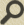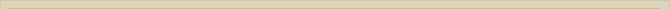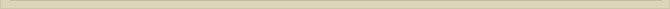## Incompetent Science – Part 2/3

When you are interested in physics you must read “Unbelievable“!

Before Einstein “discovered” relativity, physical science concluded that the “field momentum” of a moving charge could not explain the mass of the electron (R.P. Feynman, Lectures on Physics Volume II, chapter 28-2 and 28-3). The false assumption that the electromagnetic mass of a charged bulb cannot explain the mass of the electron results in the addition of a “mechanical” mass.

The article The Equivalence of Kinetic and Magnetic Energy (Galilean Electrodynamics 17, 110-114 November/December 2006) proves that the above mentioned mathematical derivation of the electromagnetic mass is invalid since the conservation law of energy is violated when that mass is calculated. This omission casts very serious doubt on Quantum Mechanic’s treatment of (charged) particles and its ability to “explain” the electron and other particles within electromagnetic theory. Attempts to modify Maxwell’s theory of electrodynamics failed dramatically (R.P. Feynman, Lectures on Physics Volume II, chapter 28-4 and 28-5).

The positivistic attitude of science elevates the empirical science of QM to a theoretical absolute. The empirical mathematical solutions of QM are considered to be the exact truth! Scientists, science journalists, referees and editors are again not prepared to consider the possible consequences of this very early omission that shapes the perspective of QM so dramatically. They reject the above paper on magnetic energy also on the argument that it is not topical; not relevant.

How could an omission that shaped physical science so profoundly ever be not topical?

Comment added November 2007: the falsification of QM

QM assumes that electrostatic-, electromagnetic- and magnetic fields are conservative. One of the implications of this assumption is that these fields must be radial. In the above mentioned article it is proven that this assumption is physically incorrect, because with the premise that the fields are conservative the conservation law of energy is violated. (Section 4. “The Electromagnetic Mass”).

When J.J. Thomson (1881) derived that the EM-theory could not explain the electromagnetic mass of an electron, during which he brutally violated the energy conservation law, no one questioned the correctness of his conclusions. His false analysis was completely copied QM and serves them as proof that the EM-theory is inadequate to describe elementary particles. Feynman copied Thomson’s mistake in his Lectures on Physics to which article I refer above.

Why did QM-physicists never question Thomson’s fundamental false derivation?

The answer to this question is to my knowledge the relentless faith of physicists in Maxwell’s equations.

Maxwell’s equations describe the assumed interrelationship between the electric field, the magnetic fieldelectric charge and electric current. Although Maxwell’s equations are mathematically correct, apparently no physicist ever verified whether these equations are also valid in physical sense!

The consequence of the energy conservation violation is that QM assumes fundamentally false EM-properties for all elementary particles.

All formulas/equations of QM/QED/QCD are based on the assumption that these fields are conservative and therefore radial. When it is proven that this assumption or premise is false all formulas/equations of QM/QED/QCD lose their theoretical significance, because all the derived particle formulas of QM/QED/QCD are physically impossible and therefore theoretically false. The derived QM-particles equations only possess mathematical/experimental significance. The QM-particles cannot exist in reality because their assumed physical properties violate the most important physics law: the energy conservation law.

QM states that it is the most successful science ever. The empirical/mathematical significance of QM is undisputable. An empirical/mathematical derived formula that describes a particle doesn’t imply that the equation is correct in physical sense. It is proven in the above mentioned article that the mathematical presentations of the QM-formulas are physically false.

The consequence of this article for QM is that QM-scientists are not allowed to deduce theoretical conclusions based on these formulas. To be able to deduct valid theoretical conclusions the formulas must also be valid in physical sense.

The above mentioned “radial” aspect of the electromagnetic field is just one of the physical entities “fields” must possess to qualify to be “conservative”. Further analysis of this violation of the energy conservation law QM demonstrates that the Maxwell equations are physically incorrect and there the theoretical basis for QM/QED/QCD collapses entirely.

Scientists cannot believe that QM is invalid. They argue that it would otherwise be impossible to derive such cunning formulas. They say the experimental proof of QM overrules the theoretical flaws! For a theoretical science the fundamental laws must always be the basics. A science degrades to a pseudo-science when basic theoretical fundamentals are ignored.

The reason why QM is empirically so successful is that thousands of scientists search every day for many years for formulas that fit the experiments. Statistically stunning formulas have to be found. Statistical “Luck” is the basis for QM’s experimental success; not theoretical insight. Still claiming that QM is a theoretical science degrades it to pseudo-science.

I tried to communicate with many expert scientists about these theoretical inconsistencies but no one was willing to discuss them.

For those who want to read the correspondence with Nobel laureate Prof. Dr. G. ‘t Hooft read: Correspondence with ‘t Hooft.

Expert scientists know that QM is theoretically disqualified and that the famous Standard Model is merely fictional science. They do not want to discuss this because then they have to admit they have been wrong for more than 100 years, and that is too embarrassing.

Expert scientists prefer to ignore and to be dishonest.

For those who want to verify that the QM-derivation of the electromagnetic mass is fundamentally false:

Electromagnetic Mass Lectures on Physics Volume II, Feynman